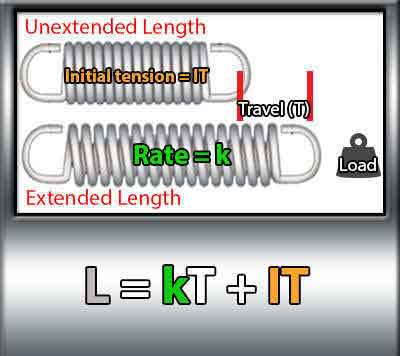<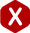# Extension Spring Design Guide

### Definition: A guide on extension and tension spring design which includes design recommendations and the equations / formulas needed to achieve a reliable extension spring design.

Need help on your extension spring design? China Spring Products Corp is here to help. Our website has many extension spring design articles which will help you in designing a reliable extension spring design. This extension spring design guide will help you by providing you with diagrams and the extension spring design formulas needed to calculate the extension spring specifications. Follow the step by step process to designing an extension spring.

## 1.) Dimensions and Material Type

### Dimensions

To begin your extension spring design, you must start setting the tolerances based on the spring’s surroundings both physical and environmental. Let’s begin with the physical dimensions. In this case we must consider the outer diameter, length inside hooks, and hook gap (hook type). Measure the space provided for the spring to fit into, also measure the distance between the mounting pieces where the extension spring shall be hooked too consider the length inside hooks. It is best for the extension spring to be pre-loaded so try to make the length inside hooks a little bit smaller for these cases. The extension spring design formulas to calculate physical dimensions are provided below as well as how to choose your material type.

### Hook Type

There are several types of extension spring hooks. The most popular ones are machine hooks, cross over center hooks, side hooks, or no hooks at all. Changing the hook type on a spring may change the coil count therefore affecting the spring’s force due to the hook lengths being different.

#### Machine Hooks & Cross-Over Center Hooks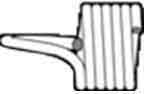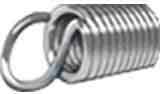#### No Hooks & Side Hooks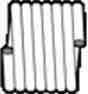### Material Type

Choosing the material type of your spring is a very important extension spring design procedure since choosing the wrong material type can cause unnecessary costs or for your spring to not work correctly. Unnecessary costs are caused when you select an exotic type of spring wire when it isn’t really necessary, and your extension spring may not work correctly if you didn’t select a material type which could withstand the environment your spring is required to resist. This means that you must consider the environment of your spring including the temperature and other conditions like moisture or corrosive areas.

## How to Measure Extension Springs

### (Physical Dimensions)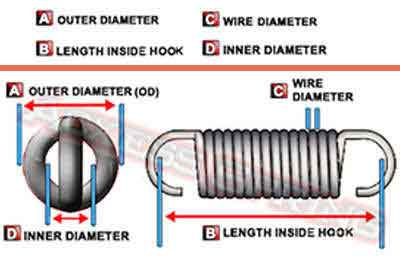### Extension Spring Design Guide Equations

#### Outer Diameter

The outer diameter (coil width) of your extension spring is calculated by adding two wire diameters to the inner diameter. You must measure the space that will be surrounding the spring’s body to set this tolerance.

2 Wire Diameters + Inner Diameter = Outer Diameter

2WD + ID = OD

#### Length Inside Hooks

The length inside hooks is calculated by either measuring the full spring outside hook to outside hook and subtracting two wire diameters or by adding the hook lengths to the body length. Remember That the hook lengths are measured from within the inner diameter of the hooks.

Overall Length – 2 Wire Diameters = Length Inside Hooks

OL – 2WD = LIH

and

Body Length + 2 Hook Lengths = LIH

BL + 2HL = LIH

When it comes to load and travel, you must understand that your spring will have a elastic limit of extension before taking a permanent set. This means that there is a maximum load and maximum safe travel. You can find out which are your spring’s maximum load possible considering hook stress as well as maximum safe travel considering hook stress by using our online extension spring calculator. The formulas to calculate your extension spring’s working loads are provided below.

### Extension Spring Design Guide Formulas

To calculate spring load (L), you must multiply your spring’s rate (k) by the distance your spring is required to travel or extend (T) and add the initial tension (IT).

Load = Rate x Travel + Initial Tension

L = kT + IT

#### Calculate Rate

There are other formulas you can follow to calculate the required rate as well as travel by using the previous formula. To calculate rate (k) you must subtract the initial tension (IT) from the load (L) and divide the result by the distance traveled (T).

Rate = (Load – Initial Tension) ÷ Travel

k = (L – IT) ÷ T

#### Calculate Travel

On the other hand, to calculate the distance traveled (T) you will achieve under a certain load and spring rate, you must again subtract the initial tension (IT) from the load (L) and divide the result by the spring rate (k).

Travel = (Load – Initial Tension) ÷ Rate

T = (L – IT) ÷ k

### We Recommend

Since there are many spring specifications to be considered when designing an extension spring, it is recommended that you speak to a spring technician or engineer to proof your design. After all, we have many years of experience working with extension spring designs like yours so feel free to contact us. It is also useful to proof or improve a design using our spring calculator, Spring Creator either online or by downloading the app to your mobile devices in case you may be on the go.

## Extension Spring Formula Guides

### Formulas for Spring Constant

#### G = psi * 10^6

Formula Key

• G = Shear Modulus of Torsion
• d = Wire size
• D = Mean Diameter
• N = Number of active coils
• Rf = Rate of extension spring (in lbs./inch)
• S = Stress (lbs. /Sq. Inch)
• M = Moment (inch-lbs.)
• D / d = Index correction (developed from Wahl Factor)

### Formula for Initial Tension

#### P = Sd^3 / 2.55D = Load in lbs

Formula Key

• d = Wire size
• D = Mean Diameter
• N = Number of active coils
• Rf = Rate of extension spring (in lbs./inch)
• S = Stress (lbs. /Sq. Inch)